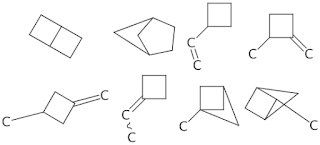Skip to main content

### Seeds and Weeds : Good/Bad Lists in Structure Generation

With the recent revival of the moleculegen (AMG) project, I've started to properly think beyond just simple generation of spaces from a formula. For example, there are 4 trillion or so C30H62 structures - which might take ... a while.

In any case, one useful feature would be to have good/bad lists of substructures (or 'nice' vs 'naughty' as I've been thinking of them. One simple approach I thought might work is to just start with the largest good substructure and generate from there. This can work :

C9H16 from 6-cycle

(By-the-way : all images done with John May's new Depict utility! It's wonderful to use :)

Which is great! Except that it doesn't always work. The problem is that leaves on the tree with a particular substructure might not have that substructure as a common parent in the tree. Consider these C6H8 structures generated from a 4-cycle:C6H10 from 4-cycle
These are not all of them. Now we filter out using subgraph isomorphism (Asad's SMSD code) from the whole space:Whole C6H10 space filtered by 4-cycle
So we get half of them by growing from a 'seed'. Now to think about filtering out the weeds...

### How many isomers of C4H11N are there?

One of the most popular queries that lands people at this blog is about the isomers of C4H11N - which I suspect may be some kind of organic chemistry question on student homework. In any case, this post will describe how to find all members of a small space like this by hand rather than using software.

Firstly, lets connect all the hydrogens to the heavy atoms (C and N, in this case). For example:

Now eleven hydrogens can be distributed among these five heavy atoms in various ways. In fact this is the problem of partitioning a number into a list of other numbers which I've talked about before. These partitions and (possible) fragment lists are shown here:

One thing to notice is that all partitions have to have 5 parts - even if one of those parts is 0. That's not strictly a partition anymore, but never mind. The other important point is that some of the partitions lead to multiple fragment lists - [3, 3, 2, 2, 1] could have a CH+NH2 or an NH+CH2.

The final step is to connect u…

### The Gale-Ryser Theorem

This is a small aside. While reading a paper by Grüner, Laue, and Meringer on generation by homomorphism they mentioned the Gale-Ryser (GR) theorem. As it turns out, this is a nice small theorem closely related to the better known Erdős-Gallai (EG).

So, GR says that given two partitions of an integer (p and q) there exists a (0, 1) matrixA iff p*dominatesq such that the row sum vector r(A) = p and the column sum vector c(A) = q.

As with most mathematics, that's quite terse and full of terminology like 'dominates' : but it's relatively simple. Here is an example:

The partitions p and q are at the top left, they both sum to 10. Next, p is transposed to get p* = [5, 4, 1] and this is compared to q at the bottom left. Since the sum at each point in the sequence is greater (or equal) for p* than q, the former dominates. One possible matrix is at the top left with the row sum vector to the right, and the column sum vector below.

Finally, the matrix can be interpreted as a bi…

### Havel-Hakimi Algorithm for Generating Graphs from Degree Sequences

A degree sequence is an ordered list of degrees for the vertices of a graph. For example, here are some graphs and their degree sequences:

Clearly, each graph has only one degree sequence, but the reverse is not true - one degree sequence can correspond to many graphs. Finally, an ordered sequence of numbers (d1 >= d2 >= ... >= dn > 0) may not be the degree sequence of a graph - in other words, it is not graphical.

The Havel-Hakimi (HH) theorem gives us a way to test a degree sequence to see if it is graphical or not. As a side-effect, a graph is produced that realises the sequence. Note that it only produces one graph, not all of them. It proceeds by attaching the first vertex of highest degree to the next set of high-degree vertices. If there are none left to attach to, it has either used up all the sequence to produce a graph, or the sequence was not graphical.

The image above shows the HH algorithm at work on the sequence [3, 3, 2, 2, 1, 1]. Unfortunately, this produce…